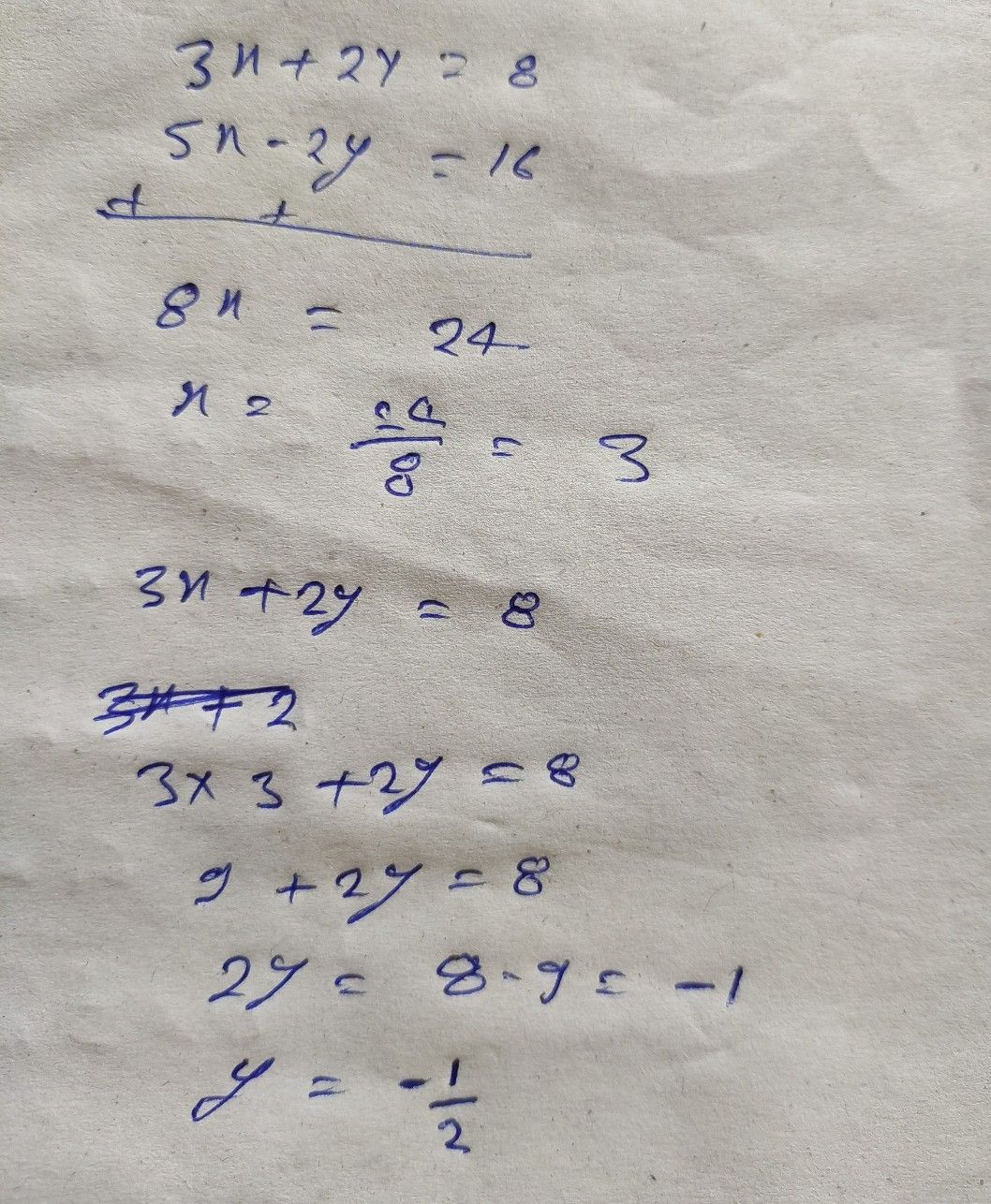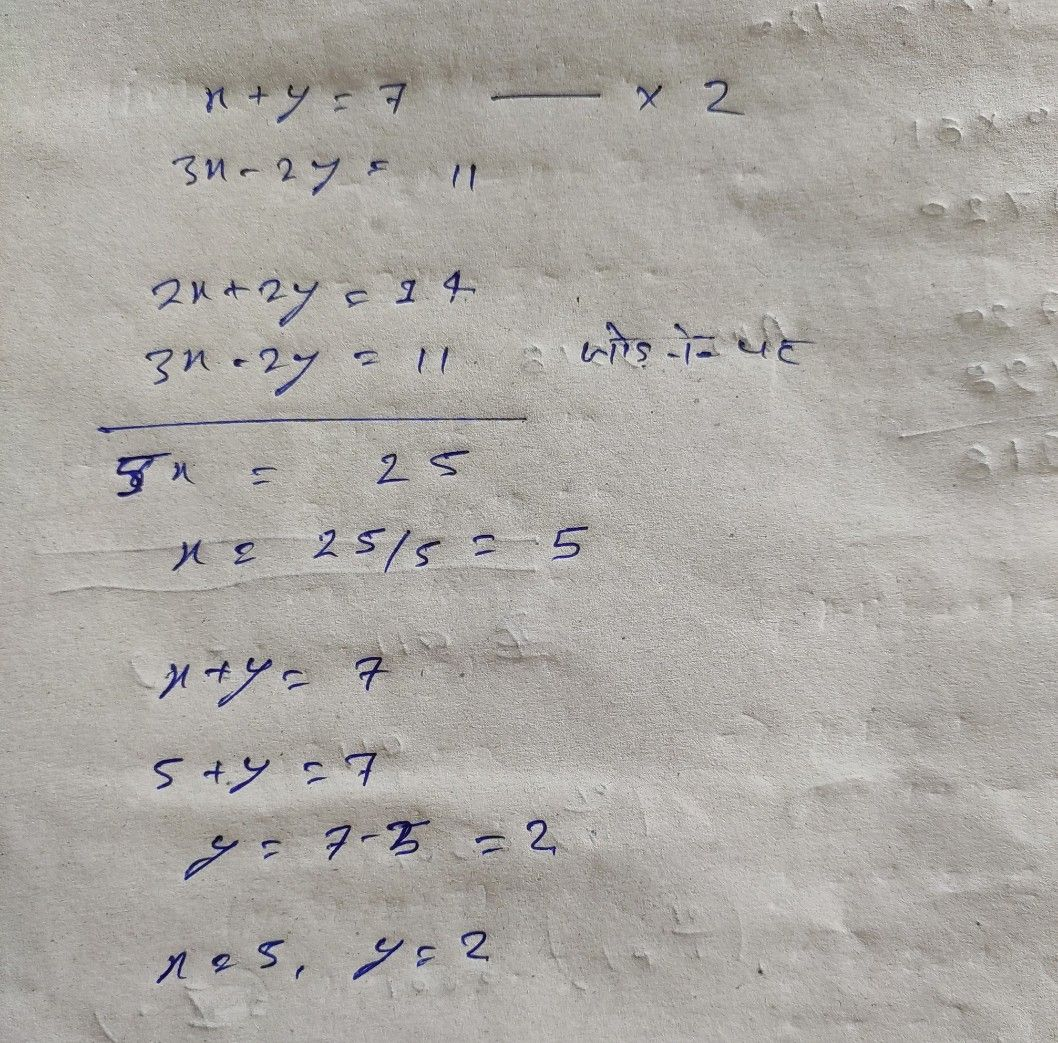Symbol
Probleml la $ABC$ $x=301$ $3x+2y=8$ $5x-2y=16$ $x.r>$ $02=$ $7$ $\pi xx$ $3x-2y=11$ $121$
10th-13th grade
Other
SolutionQanda teacher - mathelian